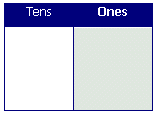# Ones and Tens

##### Math Lesson Plan
Objectives
Students will be able to recognize the relationship between ones and tens.Materials
place value mats (ones and tens only), straws, rubber bands

Procedure
1. This activity works best if preceded by an activity dealing with digit place in general, such as the lesson plan in this section titled "Digit Place." If you do such an activity, refresh the class' memory about what they learned during that activity.
2. Pass out place value mats and straws. Explain the materials to the class or group you are working with.
3. Count to 10 with them while they place one straw in the ones column for each count. Clap on the count of 10.
4. Have students group the straws with a rubber band and move the group to the tens column. Discuss how ten ones make one ten.
5. Write the numbers 10 and 20 on the board and discuss the relationship between the number of groups of ten in each number.
6. Repeat the place value mat exercise with 20, clapping on 10 and 20.
7. Continue this exercise with various numbers. You may want to use number cards that students can place above each column to show the number that their work mats show. This will help to reinforce the concept of moving from the ones to the tens column after ten straws have been counted.

Closure
Have students work together to show certain numbers on their charts. After working with the multiples of ten, try other 2-digit numbers.

Evaluation
Were children able to understand the concept that 10 ones make one ten? Did they have to continuously count one by one or did they just put a straw in the tens column to represent ten after a while?